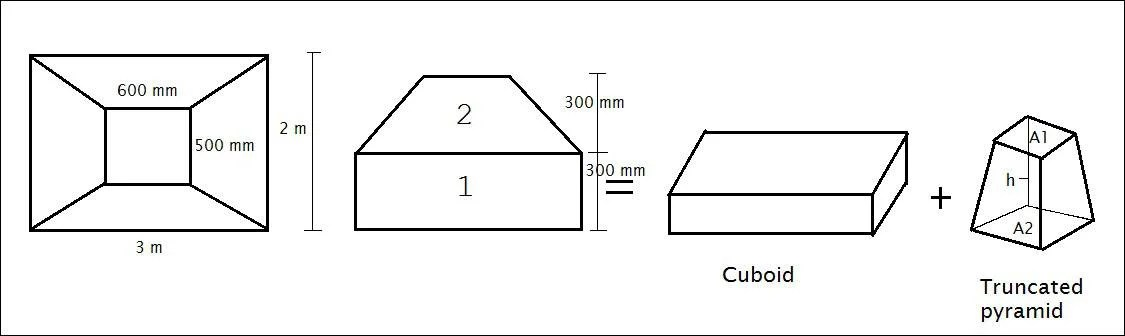## Register Now

Lorem ipsum dolor sit amet, consectetur adipiscing elit.Morbi adipiscing gravdio, sit amet suscipit risus ultrices eu.Fusce viverra neque at purus laoreet consequa.Vivamus vulputate posuere nisl quis consequat.

## How To Calculate Concrete Volume For Trapezoidal Footing/Sloped Footing

How To Calculate Concrete Volume For Trapezoidal Footing:

A trapezoid is a combination of a cuboid and a truncated pyramid.

Trapezoidal footing = Cuboid + trancated pyramid.

So to calculate the volume of trapezoidal footing we need to calculate the volume of a cuboid and a truncated pyramid.1. Volume of  Rectangular Cuboid = length x Breadth x Height = 3 x 2 x 0.3 = 1.8 m³

2. Volume of Truncated pyramid = 1/3 (A1 + A2 + √A1A2) x h

Where A1 = Area of the top base.

A2 = Area of bottom base.

A1 = 0.6 x 0.5 = 0.3 m²

A2 = 3 x 2 = 6 m²

Volume of truncated pyramid = 1/3(0.3 + 6 + √0.3×6) x 0.3 = 0.81 m³

∴ Total volume of trapezoidal footing = Volume of Cuboid + Volume of Truncated pyramid

= 1.8 + 0.81 = 2.61 m³.

So total concrete volume for trapezoidal footing is 2.61 m³.